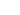# COUPON Function

This article explains how to use COUPON function

# Syntax

``COUPON(equation, coupon_question_data)``
• `Equation` - Formula from the main equation in which you want to apply the discount. The equation can be a fixed
• `Coupon_question_data` - coupon data object.

# Sample usage

``COUPON(100, QA{data})``

Or

``COUPON(VA, QC{data})``

Note: you can obtain the coupon question data by referencing the question (e.g. QB) directly followed by the data reference in curly brackets (e.g.{data}) = QB{data}

# Examples

We have a formula for a “Total price”: `QA` + `QB`

• Total price result is: \$100
• We want to add 10% off, so we add a coupon item (`QC`) and set up the coupon configuration.

The function of the “total price” is:

``COUPON(QA + QB, QC{data})``

“Total price” with coupon included is \$90